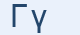Greek Letter Gamma (Γάμα - Γ)"Gamma" is the same word as "camel", in Semitic "gimel". The letter looks like a camel's head. This letter passed into the Latin alphabet in this position in a curved form as "C". The Etruscans did not distinguish between the "g" and "k" sounds, so Greek gamma came to have the "k" sound in Latin. The Romans needed another letter for the "g" sound, so they added a bar to the "C" to get "G".

"Γ"Letter in mathematics and in science

Γ represents:

• the gamma function, a generalization of the factorial
• the upper incomplete gamma function
• the gamma distribution, a continuous probability distribution defined using the gamma function
• the Christoffel symbols of the second kind

γ represents:

• the lower incomplete gamma function
• the third angle in a triangle
• the Euler-Mascheroni constant in mathematics.
• second-order sensitivity to price in mathematical finance
• a gamma ray
• the heat capacity ratio in thermodynamics
• the Lorentz factor in special relativity

Greek words with "γ"

γυρίζω, γάτα